﻿ 高压捕获翼前缘型线优化和分析文章快速检索 高级检索
 力学学报2016, Vol. 48Issue (4): 877-885  DOI: 10.6052/0459-1879-16-0360

### 引用本文 [复制中英文]

[复制中文]
Li Guangli , Cui Kai , Xiao Yao , Xu Yingzhou . LEADING EDGE OPTIMIZATION AND PARAMETER ANALYSIS OF HIGH PRESSURE CAPTURING WINGS[J]. Chinese Journal of Ship Research, 2016, 48(4): 877-885. DOI: 10.6052/0459-1879-16-036.
[复制英文]

### 文章历史

2016-01-27 收稿
2016-05-10网络版发表

0 引言

1 基准构型简介及性能评估 1.1 基准构型简介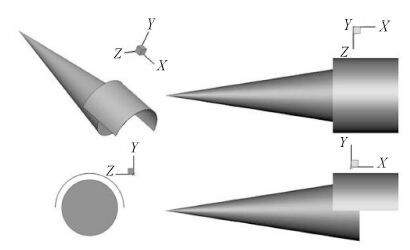图 1 基准构型三维效果图和三视图 Fig. 1 Three dimensional and three-view of the baseline configuration
1.2 基准构型性能评估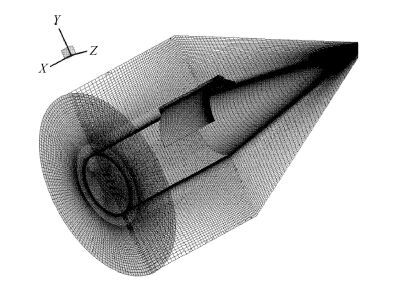图 2 基准构型计算网格示意图 Fig. 2 Illustration of computational grid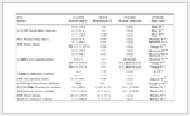表 1 基准构型各部件升阻力系数及升阻比 Table 1 Aerodynamic coefficients of the baseline configuration
2 优化设计流程及方法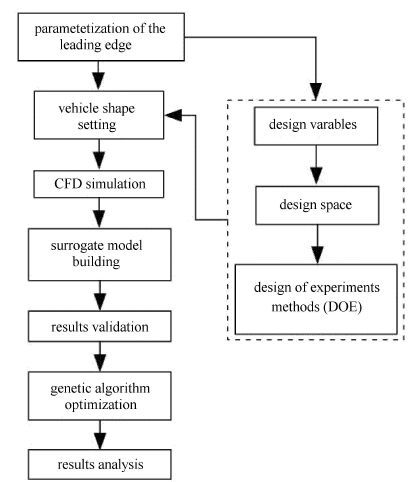图 3 优化设计流程图 Fig. 3 Flowchart of the optimization
2.1 捕获翼参数化设计方法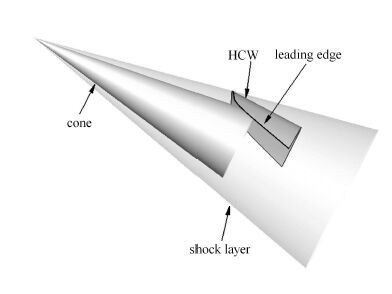图 4 锥体组合捕获翼构型设计图示 Fig. 4 Illustration of the design of cone combination with HCW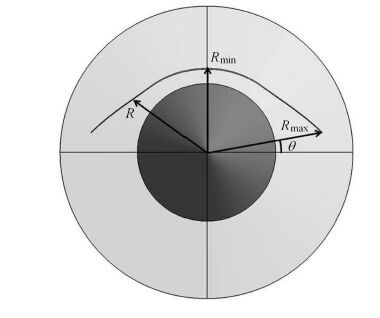图 5 前缘线控制参数 Fig. 5 Control parameters of the leading edge along the flow direction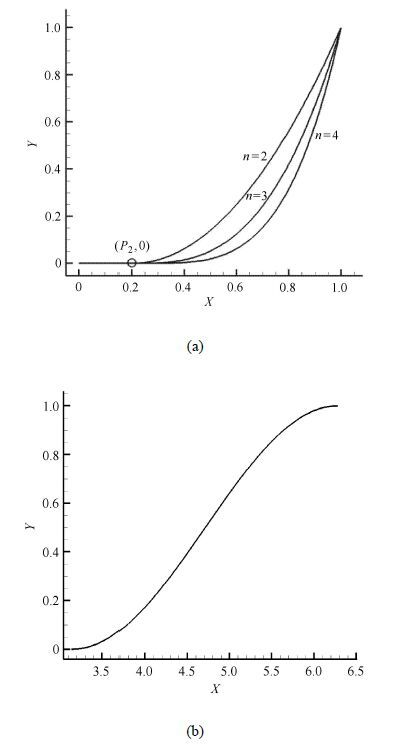图 6 幂次(a)和余弦(b)控制函数曲线 Fig. 6 Curves of the power (a) and cosine (b) control function
 \eqalign{ & R\left( {{P_1},{P_2},n} \right) = \cr & {R_{\min }} + ({R_{\max }} - {R_{\min }})\left[ {{P_1}{f_1}({P_{2,}}n) + (1 - {P_1}){f_2}} \right] \cr} (1)
 $${f_1}({P_{2,}}n) = \left\{ {\matrix{ {0,t \in [0,{P_2}]} \cr {{{\left( {{{t - {P_2}} \over {1 - {P_2}}}} \right)}^n},t \in ({P_2},1)} \cr } } \right.$$ (2)
 $${f_2} = {{\cos \alpha + 1} \over 2},\alpha \in \left[ {\pi ,2\pi } \right]$$ (3)表 2 设计变量取值范围 Table 2 Boundary values of the design space
2.2 均匀实验设计方法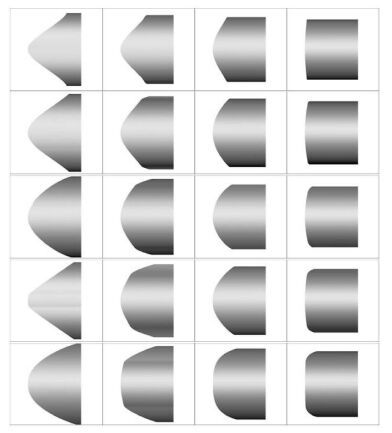图 7 构型样本集 Fig. 7 Sample set of configurations

2.3 代理模型构建

 $$y\left( x \right) = {a_0} + \sum\limits_{i = 1}^n {{a_i}} {x_i} + \sum\limits_{\matrix{ {i = 1} \cr {j = 1} \cr } }^n {{a_{ij}}} {x_i}{x_j}$$ (4)

 $$y(x) = \sum\limits_{i = 1}^P {{w_i}} \cdot \varphi \left( {\left\| {x - {x_i}} \right\|} \right)$$ (5)
 $$\varphi (\left\| {x - {x_i}} \right\|) = \varphi (r) = \exp ( - {{{r^2}} \over {2{\delta ^2}}})$$ (6)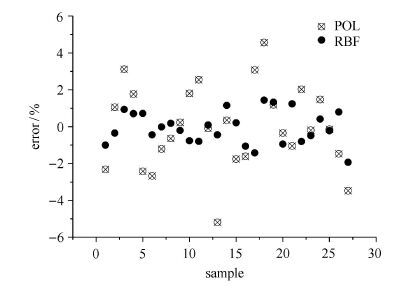图 8 多项式(POL)和径向基函数(RBF)代理模型精度对比 Fig. 8 Precision comparison between the polynomial model (POL) and the RBF model (RBF)
2.4 优化目标和方法

3 优化结果与分析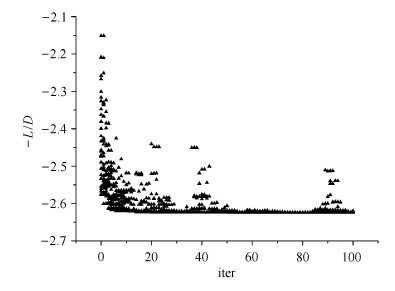图 9 目标函数收敛曲线 Fig. 9 The convergence history of the objective function表 3 优化外形和基准外形气动参数对比 Table 3 Comparison of the aerodynamic parameters between the optimal and the baseline configurations表 4 基准外形与优化外形的设计参数比较 Table 4 Comparison of the design parameters between the baseline and the optimal configurations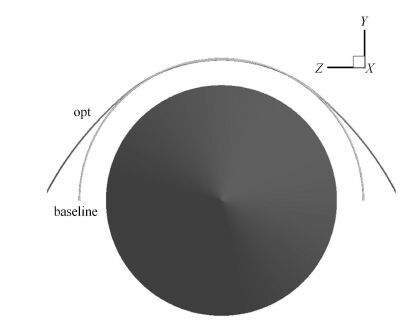图 10 优化外形和基准外形比较(轴向) Fig. 10 Shape comparison between the optimal and the baseline configurations (in axial direction)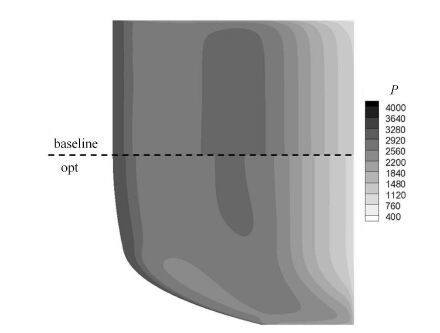图 11 优化外形与基准外形下表面压力云图对比 Fig. 11 Pressure contours comparison between the optimal and the baseline configurations (on the lower surface)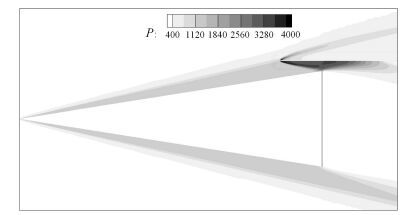图 12 优化外形对称面压力分布云图 Fig. 12 The pressure contours in the symmetry plane of the optimal configuration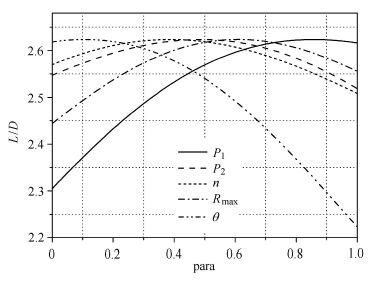图 13 升阻比与设计参数的变化曲线 Fig. 13 Curves of the $L/D$ versus each design parameter

4 结论

  Kuchemann D. The Aerodynamic Design of Aircraft[M]. Oxford: Pergamon Press, 1978 . (0)  叶友达. 近空间高速飞行器气动特性研究与构型设计优化[J]. 力学进展,2009, 39 (6) : 683-694. ( Ye Youda. Study on aerodynamic characteristic and design of optimization for high speed near space vehicles[J]. Advances in Mechanics,2009, 39 (6) : 683-694. (in Chinese) ) (0)  Sullivan RB, Winters B. X-34 program overview. AIAA Paper 1998-3516, 1998 (0)  Jazra T, Preller D, Smart MK. Design of an airbreathing second stage for a rocket-scramjet-rocket launch vehicle[J]. Journal of Spacecraft and Rockets,2013, 50 (2) : 411-422. DOI: 10.2514/1.A32381. (0)  Walker S, Sherk J, Shell D, et al. The DARPA/AF Falcon Program: The Hypersonic Technology Vehicle #2 (HTV-2) flight demonstration phase. AIAA Paper 2008-2539, 2008 (0)  Walker S, Tang M, Mamplata C. TBCC propulsion for a mach 6 hypersonic airplane. AIAA Paper 2009-7238, 2009 (0)  Nonweiler TRF. Delta wings of shapes amenable to exact shockwave theory[J]. Journal of the Royal Aeronautical Society,1963, 67 : 39-40. DOI: 10.1017/S0368393100090027. (0)  崔凯, 杨国伟. 基于CFD 分析的弧形翼导弹气动外形优化[J]. 中国科学:物理学力学天文学,2009, 39 (6) : 865-873. ( Cui Kai, Yang Guowei. Shape optimization for hypersonic arc-wing missiles based on CFD[J]. Sci Sin-Phys Mech Astron,2009, 39 (6) : 865-873. (in Chinese) ) (0)  胡守超, 崔凯, 李广利, 等. 基于DOE 方法的高超声速飞机前缘型线优化分析[J]. 力学学报,2015, 48 (2) : 290-299. ( Hu Shouchao, Cui Kai, Li Guangli, et al. Optimization and analysis of the leading edge shape for hypersonic airplanes based on DOE methods[J]. Chinese Jounal of Theoretical and Applied Mechanics,2015, 48 (2) : 290-299. (in Chinese) ) (0)  Mangin B, Benay R, Chanetz B, et al. Optimization of viscous waveriders derived from axisymmetric power-law blunt body flows[J]. Journal of Spacecraft and Rockets,2006, 43 (5) : 990-998. DOI: 10.2514/1.20079. (0)  Lobbia M, Suzuki K. Multidisciplinary design optimization of hypersonic transport configurations using waveriders. AIAA Paper 2014-2359, 2014 (0)  Graves R. Continuum-limit design and optimization of an osculating-cones waverider at Mach 20. AIAA Paper 2013-1096, 2013 (0)  Lobbia M. Optimization of waverider-derived crew reentry vehicles using a rapid aerodynamics analysis approach. AIAA Paper 2015-0757, 2015 (0)  Knittel J, Lewis M. Multidisciplinary optimization of starbody waverider shapes for lifting aerocapture with orbital plane change. AIAA-2012-5809, 2012 (0)  张锋涛, 崔凯, 杨国伟, 等. 基于神经网络技术的乘波体优化设计[J]. 力学学报,2009, 41 (3) : 418-424. ( Zhang Fengtao, Cui Kai, Yang Guowei, et al. Optimization design of waverider based on the artificial neural networks[J]. Chinese Jounal of Theoretical and Applied Mechanics,2009, 41 (3) : 418-424. (in Chinese) ) (0)  Cui K, YangGW. The effect of conical flowfields on the performance of waveriders at Mach 6[J]. Chinese Science Bulletin,2007, 52 (1) : 51-64. (0)  朱旭程, 侯志强, 李日华. 基于样条曲线的乘波体外形优化方法[J]. 空气动力学学报,2007, 25 (3) : 396-399. ( Zhu Xucheng, Hou Zhiqiang, Li Rihua. Spline-based waverider configuration optimization method[J]. Acta Aerodynamica Sinica,2007, 25 (3) : 396-399. (in Chinese) ) (0)  刘济民, 侯志强, 宋贵宝, 等. 高超声速巡航导弹乘波体构型优化设计与性能分析[J]. 空气动力学学报,2011, 29 (1) : 118-123. ( Liu Jimin, Hou Zhiqiang, Song Guibao, et al. Optimization design and aerodynamic characteristic analysis of waverider based hypersonic cruise missile configuration[J]. Acta Aerodynamica Sinica,2011, 29 (1) : 118-123. (in Chinese) ) (0)  Bowcutt KG, Anderson JD, Capriotti D. Viscous optimized hypersonic waveriders. AIAA Paper 1987-0272, 1987 (0)  Corda S, Anderson JD. Viscous optimized hypersonic waveriders designed from axisymmertirc flow fields. AIAA-88-0396, 1988 (0)  Lobbia M, Suzuki K. Experimental investigation of a Mach 3.5 waverider designed using computational fluid dynamics[J]. AIAA Journal,2014, 53 (6) : 1590-1601. (0)  高太元, 崔凯, 胡守超, 等. 高超声速飞行器上壁面多目标优化及性能分析[J]. 力学学报,2013, 45 (2) : 193-201. ( Gao Taiyuan, Cui Kai, Hu Shouchao, et al. Multi-objective optimization and aerodynamic performance analysis of the upper surface for hypersonic vehicles[J]. Chinese Journal of Theoretical and Applied Mechanics,2013, 45 (2) : 193-201. (in Chinese) ) (0)  崔凯, 胡守超, 李广利等. 尖/钝化前缘乘波体压缩面优化分析. CSTAM 2012-B03-0305 ( Cui Kai, Hu Shouchao, Li Guangli, et al. Optimization and analysis of waverider compression surface with sharp or blunted leading edges. CSTAM 2012-B03-0305 (in Chinese) ) (0)  崔凯, 李广利, 胡守超, 等. 高速飞行器高压捕获翼气动布局概念研究[J]. 中国科学: 物理学力学天文学,2013, 43 (5) : 652-661. DOI: 10.1360/132013-60. ( Cui Kai, Li Guangli, Hu Shouchao, et al. Conceptual studies of the high pressure zone capture wing configuration for high speed air vehicles[J]. Sci Sin-Phys Mech Astron,2013, 43 (5) : 652-661. (in Chinese) DOI: 10.1360/132013-60. ) (0)  Cui K, Li GL, Xiao Y. Aerodynamic performance study of high pressure capturing wing configuration. AIAA Paper 2015-3388, 2015 (0)  李广利, 崔凯, 肖尧, 等. 高压捕获翼位置设计方法研究[J]. 力学学报,2016, 48 (3) : 576-584. ( Li Guangli, Cui Kai, Xiao Yao, et al. The design method research for the position of high pressure capturing wing[J]. Chinese Journal of Theoretical and Applied Mechanics,2016, 48 (3) : 576-584. (in Chinese) ) (0)  方开泰, 刘民千, 周永道. 试验设计与建模[M]. 北京: 高等教育出版社, 2011 . ( Fang Kaitai, Liu Minqian, Zhou Yongdao. Design and Modeling of Experiments[M]. Beijing: Higher Education Press, 2011 . (in Chinese) ) (0)  Yang G, Chen D, Cui K. Response surface technique for static aeroelastic optimization on a high-aspect-ratio wing[J]. Journal of Aircraft,2009, 46 (4) : 1444-1450. DOI: 10.2514/1.42370. (0)  张来平, 马戎, 常兴华, 等. 虚拟飞行中气动、运动和控制耦合的数值模拟技术[J]. 力学进展,2014, 44 : 201410. (0)  邓磊, 乔志德, 杨旭东, 等. 基于响应面法的低速翼型气动优化设计[J]. 空气动力学学报,2010, 28 (4) : 430-435. ( Deng Lei, Qiao Zhide, Yang Xudong, et al. Aerodynamic optimization design of low-speed airfoil based on response surface methodology[J]. Acta Aerodynamica Sinica,2010, 28 (4) : 430-435. (in Chinese) ) (0)  熊伟, 张艳玲, 洪蓓, 等. 基于RBF 神经网络的轴对称飞行器弹道设计研究[J]. 导弹与航天运载技术,2014 (4) : 9-13. (0)
LEADING EDGE OPTIMIZATION AND PARAMETER ANALYSIS OF HIGH PRESSURE CAPTURING WINGS
Li Guangli, Cui Kai, Xiao Yao, Xu Yingzhou
State Key Lab of High-temperature Gas Dynamics, Institute of Mechanics, Chinese Academy of Sciences, Beijing 100190, China
Abstract: In this paper, an aerodynamic optimization is carried out to analyze the effect of leading edge shape on the aerodynamic performance for high pressure capturing wing (HCW) configurations. First, a parameterized method for the wing leading edge of an HCW is developed by combining a power function and a cosine function. The lift-to-drag ratio of the configuration is chosen as the goal of maximization. Next, a numerical optimization flow is constructed by combining with the uniform experimental design method, the computational fluid dynamics, the radial basis function surrogate model method and the genetic algorithm on the basis of the comparison of the accuracy between the polynomial surrogate model and the radial basis functional surrogate model. At last, the sensitivity analysis of optimization results for each parameter is implemented. As the comparison of the optimal to the baseline, the results show that the lift coefficient increased by 8.1%, the drag coefficient is decreased by 12.2%, the lift-to-drag ratio increased by 23.4%. In addition, the sensitivity analysis results show that the lift-to-drag ratio presents non-linear relationship with the five design parameters and the angle of wingspan had the greatest influence, followed by the exponential curve parameter, the effect of other three parameters on the lift-to-drag ratio is relatively weak.
Key words: high pressure capturing wing    computational fluid dynamic    surrogate model    shape optimization